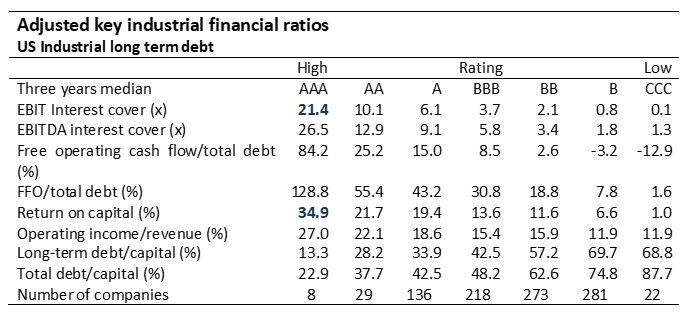## Review questions

The expected loss is defined as follows:

Expected loss = probability of default (%) x (exposure at default – value of recovery)

The probability of default is an estimate of the likelihood that the borrowing firm may default. The exposure at default is the outstanding debt including unpaid interests at default. The net proceed that the creditors receive given a default is the ultimate recovery. The recovery rate is proportion of the exposure at default which is recovered (ultimate recovery divided by exposure at default).

Use and type of loan

1. An understanding of the intended use of the loan
2. An understanding of the type of financing (loan)

Estimating probability of default

1. An understanding the business risk: an analysis of the industry, the firm and management
2. An assessment of the firm’s financial health based on historical financial data
• Financial ratios
• Credit rating
• Statistical models (multiple discriminant analysis, logit analysis etc.)
1. An assessment of the firm’s financial health based on simulation of future cash flows

Estimating exposure at default and ultimate recovery (ratio)

1. Exposure at default
2. Ultimate recovery and recovery rate

Estimating the expected loss

1. Summarising the results of the credit analysis
2. Some caveats when estimating the expected loss
• Correlation between probability of default and loss given default
• Banks loan portfolio analysis (diversification and concentration risk)
• Corporate loans
• Bonds
• Medium-term notes – similar to bonds but dealers have no underwriting obligations
• Private placements
• Convertible debt and other hybrid instruments
 Sound financing structure Liquidity reserve for rainy days Equity ratio or Financial leverage ratio Liquidity reserve ratio (Modified) risk tolerance ratio Liquidity efficiency Long-term financing coverage ratio Working capital ratio Funds from operations sufficient to pay debt Liquidity cycke (days) Interest coverage ratio (cash or EBIT) Short-term liquidity risk CFO to debt (or NIBL) ratio Current ratio or modified current ratio Debt (NIBL) to EBITDA (or CFO) ratio CFO to current financing liabilities ratio Capital expenditure ratio Cash burn rate of Liquidity reserve stress test
• Univariate analysis (e.g. Beaver 1966)
• Multiple discriminant analysis (e.g. Altman 1968)
• Logit analysis (e.g. Ohlson 1980)

They rate debt according to the underlying risk. This risk is assessed based on a variety of financial ratios as shown in the example below from Standard & Poor’s:The estimation of exposure at default is not always straightforward. Most banks take a conservative view and assume that the current debt equals exposure at default. Some banks try to estimate the exposure of default at the most critical point in time; i.e. the year where the probability for default is highest.

An estimation of the liquidation value typically follows these steps:

Book value of equity

+/– The difference between the liquidation value and book value of assets

+/– The difference between the liquidation value and book value of liabilities

+/– The liquidation value of off-balance sheet items

– Fees to lawyers, auditors, etc.

= Liquidation value

• Correlation between probability of default and loss given default
• Banks loan portfolio analysis (diversification and concentration risk)